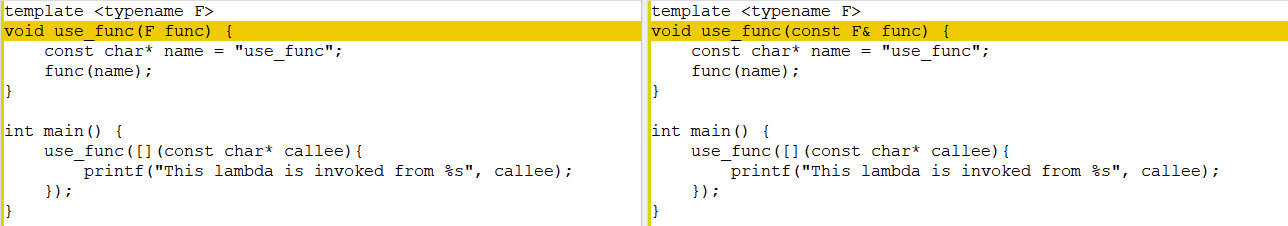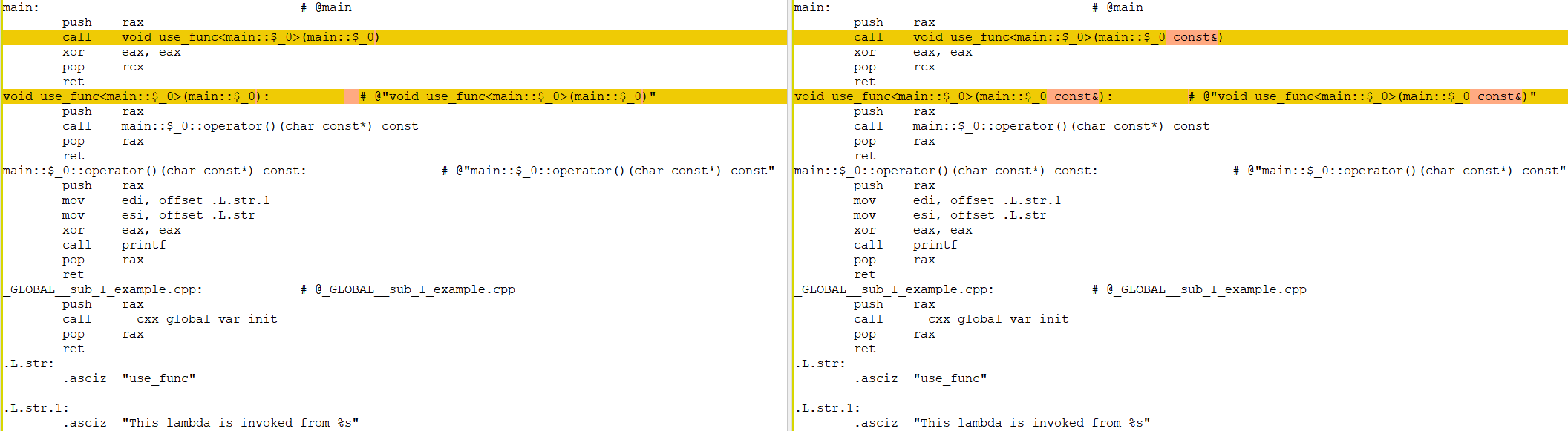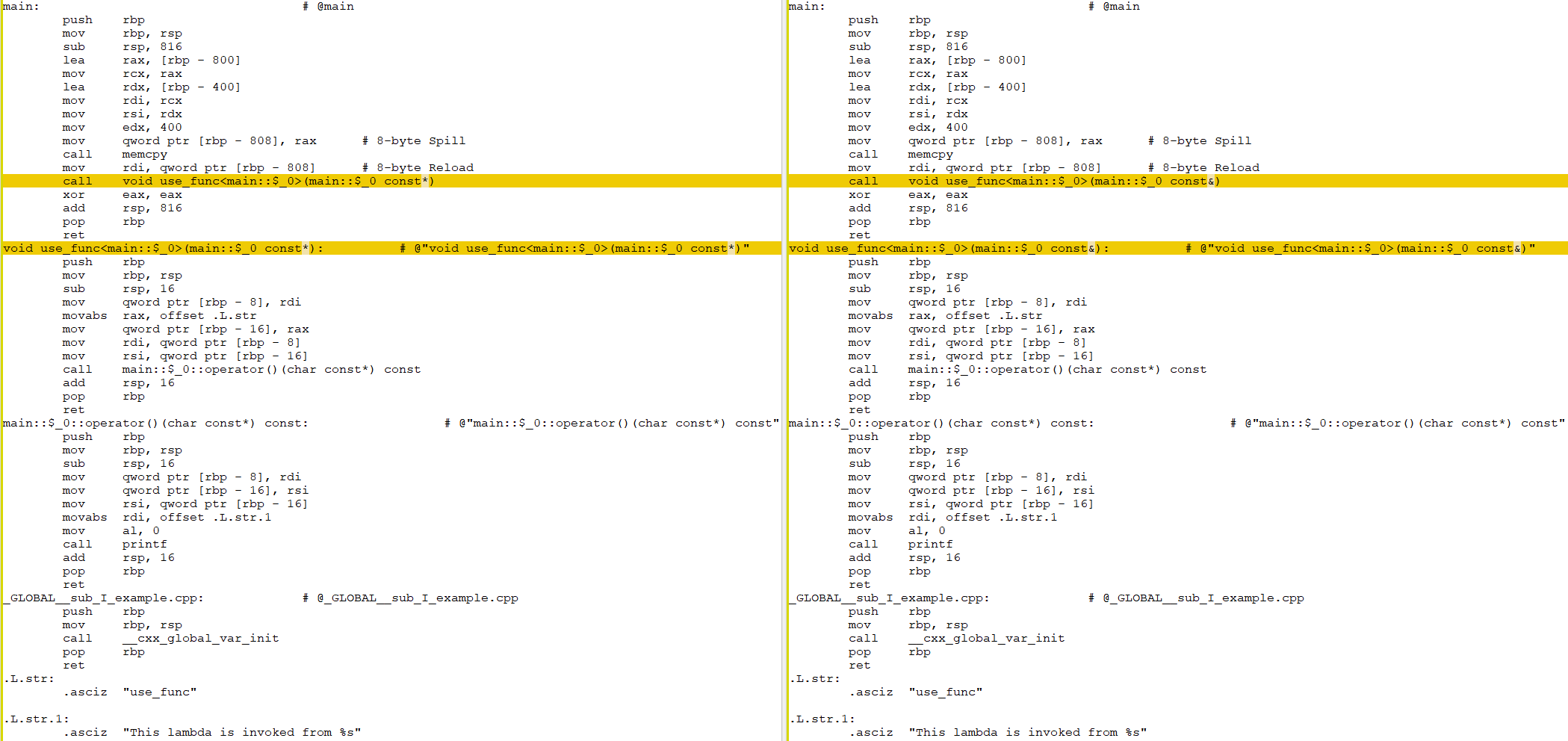# How To Pass Lambda Functions in C++ (By Value, By L-Value Reference, By Universal Reference)

A question that I’ve asked on StackOverflow: C++ best practice: Pass use-only (not stored) lambda argument to function by const-reference or by forwarding-reference (a.k.a. universal-reference) tempted me to create this post. I was looking for the best way of how to pass-in lambda functions as a parameter. There are several ways and it might not be obvious which one is the optimal way:

• by (const) l-value reference,
• by universal reference*, or
• by value?

* I will be using the term “universal reference” in this blog post and not “forwarding reference” since those references will not be forwarded in the code examples but only used in place. Therefore, good old “universal reference” seems to be the more appropriate term in this context.

## How Does The STL Do It?

A look into the STL reveals that in (almost?) all places lambdas are passed by value. The UnaryPredicate of std::find_if, for example, is passed by value in all overloads. Why might it be a good idea to pass by value?

To answer this question, let us consider a lambda which does not capture any data as shown in Code Listing 1.

auto lambda = [](const char* callee){
printf("This lambda is invoked from %s", callee);
};


Code Listing 1: A lambda that does not capture any data.

It only contains functionality, but no data. In such a case, it does not really matter how exactly we capture the lambda. No matter if we capture it by reference or by value, the compiler only has to plug in the instructions of the lambda but no data needs to be transferred, whatsoever.

We can verify this by taking a look at the generated assembly code (e.g. using COMPILER EXPLORER): The two different code versions shown in Figure 1 compile to the same assembly instructions as shown in Figure 2 (x86-64 clang 11.0.0 -O1, diff created with WinMerge).Figure 1: Two versions of a function, the left accepts a lambda by value, the right accepts it by const reference.Figure 2: The assembly code of the two versions from Figure 1 compared with each other.

Where it can make a difference is when the lambda captures data. If a lambda captures large amounts of data, that data would have to be copied whenever a lambda is passed by value. Let us assume that a lambda captures a large vector by value, as shown in Code Listing 2.

std::vector<uint8_t> blob;
auto lambda = [blob](){ };


Code Listing 2: A lambda that captures a lot of data.

In such a case, the entire vector would be duplicated every time lambda is passed by value, implying an allocation of (potentially) a lot of data.

The STL, however, obviously assumes that such cases are rare or considers them bad practice – and rightfully so! I.e. for “small” lambdas, passing by value looks like the way to go.

We can therefore state:

## Accept a Lambda By Value If It Captures Only Small Amounts of Data

For many of the STL’s functions, it can be expected that a lambda should only capture small amounts of data. But even if it requires to capture a lot of data, the cost of passing a lambda around by value can potentially be minimized. An alternative to Code Listing 2 that can be cheaply passed around by value is shown in Code Listing 3.

std::vector<uint8_t> blob;
auto lambda = [&blob](){ };


Code Listing 3: Capturing the std::vector by reference incurs the small cost of copying only one reference (which is the size of one pointer to a memory address) when passing lambda around by value. Please note, however, that this is only safe if blob outlives lambda.

## Are The Alternatives Any Good?

All the alternatives to passing a lambda by value actually capture a lambda’s address, be it by const l-value reference, by non-const l-value reference, by universal reference, or by pointer. No matter which alternative we are choosing, it results in exactly the same code as can be seen in Figure 3 which compare the generated assembly code from Code Listing 4 and Code Listing 5 (x86-64 clang 11.0.0 -O0).

template <typename F>
void use_func(const F* func) {
const char* name = "use_func";
(*func)(name);
}

int main() {
std::array<int, 100> blob;
auto lambda = [blob](const char* callee){
printf("This lambda is invoked from %s", callee);
};
use_func(&lambda);
}


Code Listing 4: Accepting a lambda by const pointer.

template <typename F>
void use_func(const F& func) {
const char* name = "use_func";
func(name);
}

int main() {
std::array<int, 100> blob;
use_func([blob](const char* callee){
printf("This lambda is invoked from %s", callee);
});
}


Code Listing 5: Accepting a lambda by const reference.Figure 3: The assembly code of Code Listing 4 and Code Listing 5 compared with each other.

So, does it even matter which one we use?

As it turns out, yes it does – but mostly usage-wise. Let’s see:

• Neither void use_func(const F* func) nor void use_func(const F& func) accept mutable lambdas.
• void use_func(F& func) does not accept temporaries. I.e. you can not invoke it like follows: use_func([](const char* callee){ });

And most interestingly, there’s also a case that the void use_func(F func) variant (i.e. the by value version that we have advocated above) can not handle, namely accepting a lambda that has captured a move-only type like std::unique_ptr – as shown in Code Listing 6 – unless you are okay with std::move-ing your lambda into the use_func, but one could argue that that is somewhat pointless since you can’t use the lambda anymore afterwards.

auto lambda = [dataPtr = std::unique_ptr<std::array<int, 100>>{}](const char* callee) {
printf("This lambda is invoked from %s", callee);
}


Code Listing 6: A move-only lambda

A lambda that contains move-only variables is only accepted by the following variants:

• template <typename F> void use_func(F& func)
• template <typename F> void use_func(F&& func)

This indicates that especially the latter variant – the one accepting a universal reference – is the most versatile one since it is the only variant which accepts all different kinds of parameters: mutable lambdas, move-only lambdas, and – of course – ordinary lambdas.

## Which Variant Shall I Use?

As reasoned in the answers and comments of the StackOverflow question mentioned initially, the by value variant is probably the one that you should default to. It might allow the compiler to optimize the code better due to fewer indirections. Data can be passed very efficiently on the stack in many cases. The fact that the STL uses these kinds of lambda parameters reinforces these points. The only real downside is that the by value-variant does not really make sense for usage with move-only lambdas.

The best alternative to accepting lambdas by value is accepting lambdas by universal reference. It has two advantages:

• Move-only lambdas can be used just fine.
• Lambdas that capture a lot of data, or that lambdas which capture variables which imply high copy costs (e.g. allocations), are handled more efficiently because the lambda (and its data) is not passed by value (therefore not leading to duplication of the lambda’s data), but only a reference to it is passed.

Tags:

Categories:

Updated: